Updating search results...

# 16 Results

View
Selected filters:
• Word Problems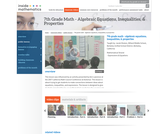Rating
0.0 stars

This lesson is about trying to get students to make connections between ideas about equations, inequalities, and expressions. The lesson is designed to give students opportunities to use mathematical vocabulary for a purpose to describe, discuss, and work with these symbol strings.The idea is for students to start gathering global information by looking at the whole number string rather than thinking only about individual procedures or steps. Hopefully students will begin to see the symbol strings as mathematical objects with their own unique set of attributes. (7th Grade Math)

Subject:
Algebra
Numbers and Operations
Material Type:
Activity/Lab
Lecture
Lesson Plan
Teaching/Learning Strategy
Provider:
Noyce Foundation
Provider Set:
Inside Mathematics
Author:
Disston, Jacob
11/30/2011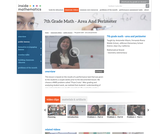Rating
0.0 stars

This lesson is based on the results of a performance task in which we realized that students' understanding of area and perimeter was mostly procedural. Therefore the purpose of this re-engagement lesson was to address student misconceptions and deepen student understanding of area and perimeter. The standards addressed in this lesson involve finding perimeter and area of various shapes, finding the perimeter when given a fixed area, and using a formula in a practical context. Challenges for our students included decoding the language in the problem and proving their thinking. (7th Grade Math)

Subject:
Mathematics
Material Type:
Activity/Lab
Lecture
Lesson Plan
Teaching/Learning Strategy
Provider:
Noyce Foundation
Provider Set:
Inside Mathematics
Author:
Villarin, Antoinette
11/30/2011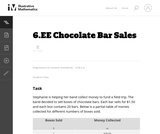Unrestricted Use
CC BY
Rating
0.0 stars

In this task students use different representations to analyze the relationship between two quantities and to solve a real world problem. The situation presented provides a good opportunity to make connections between the information provided by tables, graphs and equations.

Subject:
Mathematics
Material Type:
Activity/Lab
Provider:
Illustrative Mathematics
Provider Set:
Illustrative Mathematics
Author:
Illustrative Mathematics
08/08/2012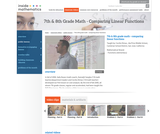Rating
0.0 stars

The foundation of this lesson is constructing, communicating, and evaluating student-generated tables while making comparisons between three different financial plans. Students are given three different DVD rental plans and asked to analyze each one to see if they could determine when the 3 different DVD plans cost the same amount of money, if ever. (7th/8th Grade Math)

Subject:
Algebra
Material Type:
Activity/Lab
Lecture
Lesson Plan
Teaching/Learning Strategy
Provider:
Noyce Foundation
Provider Set:
Inside Mathematics
Author:
Dimas, Cecilio
11/30/2011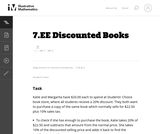Unrestricted Use
CC BY
Rating
0.0 stars

This purpose of this task is to help students see two different ways to look at percentages both as a decrease and an increase of an original amount. In addition, students have to turn a verbal description of several operations into mathematical symbols.

Subject:
Mathematics
Algebra
Material Type:
Activity/Lab
Provider:
Illustrative Mathematics
Provider Set:
Illustrative Mathematics
Author:
Illustrative Mathematics
05/01/2012Unrestricted Use
CC BY
Rating
0.0 stars

In this task students are asked to write an equation to solve a real-world problem. There are two natural approaches to this task. In the first approach, students have to notice that even though there is one variable, namely the number of firefighters, it is used in two different places. In the other approach, students can find the total cost per firefighter and then write the equation.

Subject:
Mathematics
Material Type:
Activity/Lab
Provider:
Illustrative Mathematics
Provider Set:
Illustrative Mathematics
Author:
Illustrative Mathematics
05/01/2012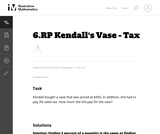Unrestricted Use
CC BY
Rating
0.0 stars

This word problem requires students to compute sales tax.

Subject:
Mathematics
Material Type:
Activity/Lab
Provider:
Illustrative Mathematics
Provider Set:
Illustrative Mathematics
Author:
Illustrative Mathematics
05/01/2012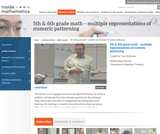Rating
3.0 stars

This lesson is a re-engagement lesson designed for learners to revisit a problem-solving task they have already experienced. Students will activate prior knowledge of graphical representations through the 'what's my rule' number talk; compare and contrast two different learners' interpretations of the growing pattern; use multiple representations to demonstrate how one of these learners would represent the numeric pattern; make connections between the different representations to more critically compare the two interpretations. (5th/6th Grade Math)

Subject:
Numbers and Operations
Material Type:
Activity/Lab
Lecture
Lesson Plan
Teaching/Learning Strategy
Provider:
Noyce Foundation
Provider Set:
Inside Mathematics
Author:
Dickinson, Fran
11/30/2011Unrestricted Use
CC BY
Rating
0.0 stars

This real world word problem requires multiple steps.

Subject:
Mathematics
Material Type:
Activity/Lab
Provider:
Illustrative Mathematics
Provider Set:
Illustrative Mathematics
Author:
Illustrative Mathematics
05/01/2012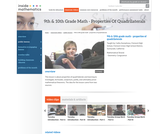Rating
0.0 stars

This lesson is about properties of quadrilaterals and learning to investigate, formulate, conjecture, justify, and ultimately prove mathematical theorems. Students will: Analyze characteristics and properties of two- and three-dimensional geometric shapes; develop mathematical arguments about geometric relationships; and apply appropriate techniques, tools, and formulas to determine measurements.Explore relationships among classes of two- and three-dimensional geometric objects, make and test conjectures about them, and solve problems involving them. Employ forms of mathematical reasoning and proof appropriate to the solution of the problem at hand, including deductive and inductive reasoning, making and testing conjectures, and using counter examples and indirect proof. Identify, formulate and confirm conjectures. Establish the validity of geometric conjectures using deduction, prove theorems, and critique arguments made by others. (9th/10th Grade Math)

Subject:
Algebra
Material Type:
Activity/Lab
Lecture
Lesson Plan
Teaching/Learning Strategy
Provider:
Noyce Foundation
Provider Set:
Inside Mathematics
Author:
Humphreys, Cathy
11/30/2011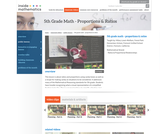Rating
0.0 stars

This lesson is about ratios and proportions using candy boxes as well as a recipe for making candy as situations to be considered. It addresses many Mathematical Reasoning standards and asks students to: Use models to understand fractions and to solve ratio problems; think about a ratio as part/part model and to think about the pattern growing in equal groups or a unit composed of the sum of the parts; find a scale factor and apply it to a ratio. (5th Grade Math)

Subject:
Ratios and Proportions
Material Type:
Activity/Lab
Lecture
Lesson Plan
Teaching/Learning Strategy
Provider:
Noyce Foundation
Provider Set:
Inside Mathematics
Author:
Lewis-Wolfsen, Hillary
11/30/2011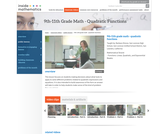Rating
0.0 stars

This lesson focuses on students making decisions about what tools to apply to solve different problems related to quadratic expressions and equations. It is also intended to build awareness of the form an answer will take in order to help students make sense of the kind of problem they are solving. (9th/10th/11th Grade Math)

Subject:
Algebra
Material Type:
Activity/Lab
Lecture
Lesson Plan
Teaching/Learning Strategy
Provider:
Noyce Foundation
Provider Set:
Inside Mathematics
Author:
Shreve, Barbara
11/30/2011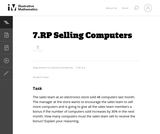Unrestricted Use
CC BY
Rating
0.0 stars

There are a couple of ways to solve this real world word problem.

Subject:
Mathematics
Material Type:
Activity/Lab
Provider:
Illustrative Mathematics
Provider Set:
Illustrative Mathematics
Author:
Illustrative Mathematics
05/01/2012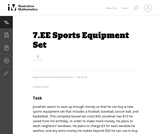Unrestricted Use
CC BY
Rating
0.0 stars

The purpose of this task is to present students with a context that can naturally be represented with an inequality and to explore the relationship between the context and the mathematical representation of that context; thus, this is an intended as an instructional task.

Subject:
Mathematics
Material Type:
Activity/Lab
Provider:
Illustrative Mathematics
Provider Set:
Illustrative Mathematics
Author:
Illustrative Mathematics
08/10/2012Unrestricted Use
CC BY
Rating
0.0 stars

This is a multi-step problem since it requires more than two steps no matter how it is solved. The problem is not scaffolded for the student, but each step is straightforward and should follow from the previous with a careful reading of the problem.

Subject:
Mathematics
Material Type:
Activity/Lab
Provider:
Illustrative Mathematics
Provider Set:
Illustrative Mathematics
Author:
Illustrative Mathematics
05/01/2012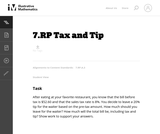Unrestricted Use
CC BY
Rating
0.0 stars

This real world word problem about calculating a tip in a restaurant requires multiple steps.

Subject:
Mathematics
Material Type:
Activity/Lab
Provider:
Illustrative Mathematics
Provider Set:
Illustrative Mathematics
Author:
Illustrative Mathematics